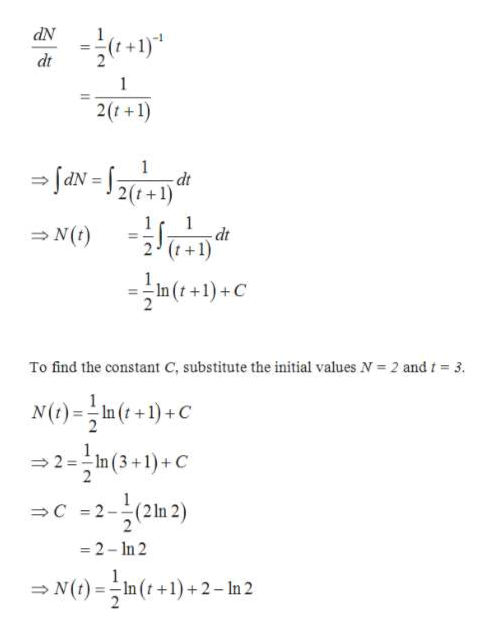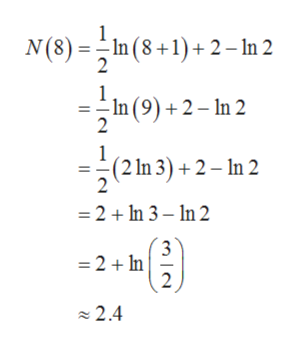# Because a new employee must learn an assigned task, production will increase with time. Suppose that for the average new employee, the rate of performance is given bydN/dt= 1/2(t+1)^-1where N is the number of units completed t hours after beginning a new task. If 2 units are completed after 3 hours, how many units are completed after 8 hours?

Question
11 views

Because a new employee must learn an assigned task, production will increase with time. Suppose that for the average new employee, the rate of performance is given by

dN/dt= 1/2(t+1)^-1

where N is the number of units completed t hours after beginning a new task. If 2 units are completed after 3 hours, how many units are completed after 8 hours?

check_circle

Step 1

To find the number of units completed in t hours by the new employee, integrate the rate of performance with respect to time as follows.help_outlineImage Transcriptionclosedt 2(t+1) dt 2(t+1) 1 dt N(t) 2 (t+1) in(+)+C To find the constant C, substitute the initial values N 2 and t 3. N()In (t+1)+C 2 2 =-In ( 3 +1)+ C C 2 21 -2-In 2 N(t)In (t+1)+2-In 2 fullscreen
Step 2

To find the number of units produced after 8 hours, substitute t = 8 in N(t).help_outlineImage TranscriptioncloseN(8)In(8+1)+2- In 2 1 In (9)+2-n 2 2 (2 1n 3)2-n 2 2 =2In 3-n 2 3 =2In 2 2.4 fullscreen
Step 3

But this is not making any sense here, as 2 units were made in 3 hours and 2.4 units in 8 hours. There is a typing mistake in the function it seems. Assuming the equation from the internet source, ...

### Want to see the full answer?

See Solution

#### Want to see this answer and more?

Solutions are written by subject experts who are available 24/7. Questions are typically answered within 1 hour.*

See Solution
*Response times may vary by subject and question.
Tagged in